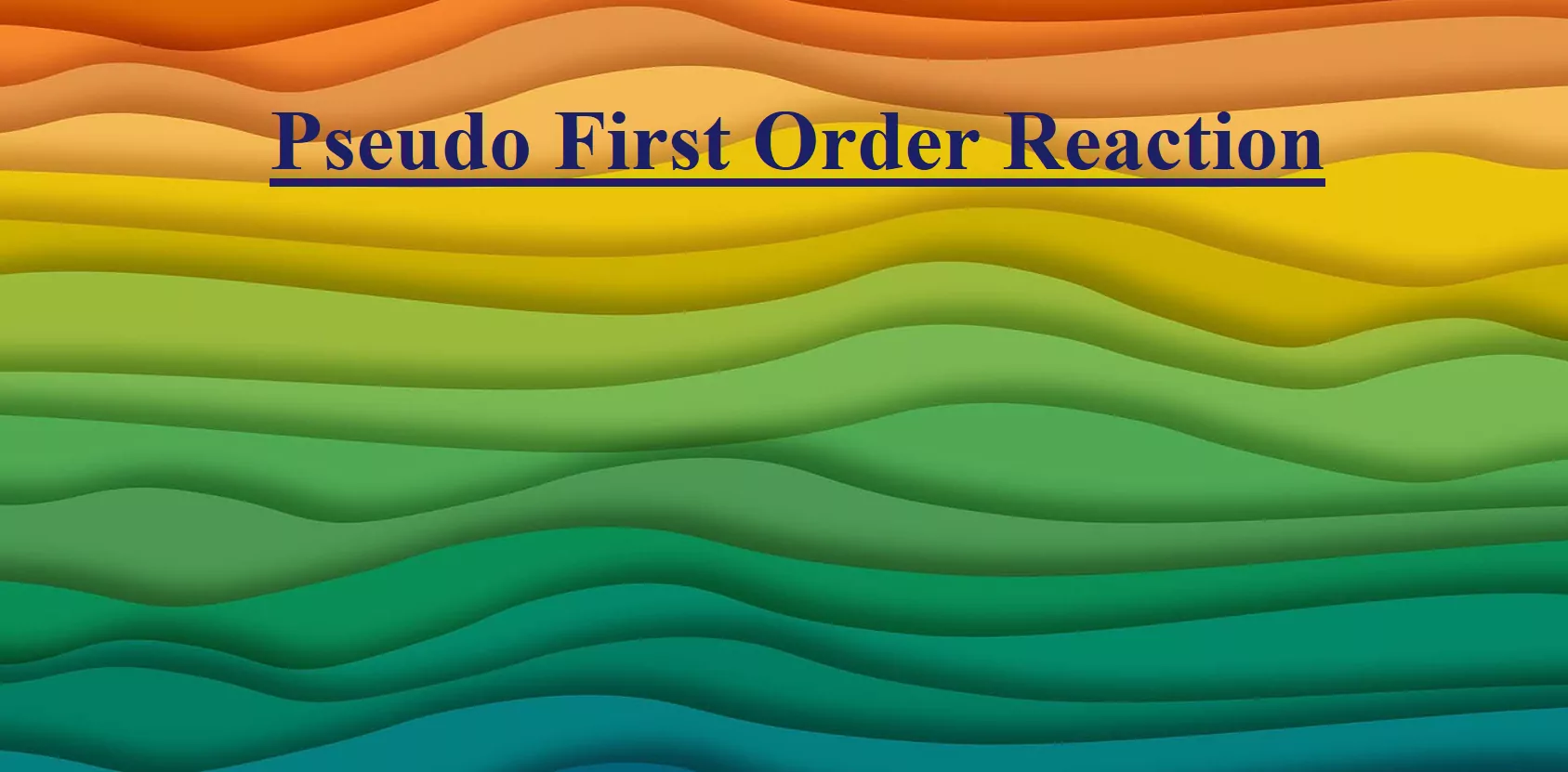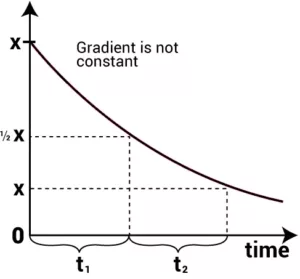# Pseudo First Order ReactionPseudo First Order Reaction

Pseudo First Order Reaction: The reactions which are not truly of first order but become reactions of first-order under certain conditions are called pseudo-first-order reactions.
Let us take a general reaction
A + B → C
The rate of reaction depends upon the concentrations of both A and B but one of the components is present in large excess and thus its concentration hardly changes as the reaction proceeds so the rate is independent of that particular component.
So, if component B is in large excess and the concentration of B is very high as compared to that of A, the reaction is considered to be a pseudo-first-order reaction with respect to A and if component A is in large excess and the concentration of A is very high as compared to that of B, the reaction is considered to be pseudo-first order with respect to B.Pseudo First Order Reaction

For example,
i) Hydrolysis of ester in acid (H+).
CH3COOC2H5 + H2O → CH3COOH + C2H5OH
Rate = k[CH3COOC2H5] [H2O]
Since water is in excess so its concentrations remain the same during the reaction and [H2O] is constant and the rate is independent of the concentration of H2O so
Rate = k[CH3COOC2H5], Where k = k[H2O]

ii) Inversion of cane sugar (in acid)
C12H22O11 + H2O → C6H12O6   +    C6H12O6
Cane sugar                   Glucose           Fructose
Since water is in excess and [H2O] is constant, so
Rate = k [C12H22O11]

(In the above reactions the concentration of H2O is in excess so the rate of reaction is independent of the concentration of water).